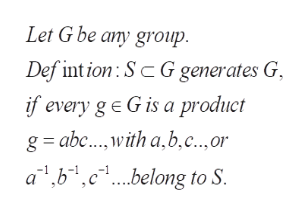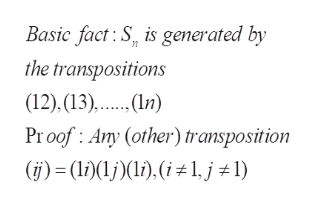Abstract AlgebraLet n ≥ 2. Show that Sn is generated by each of the following sets. (a) S1 = {(1, 2), (1, 2, 3), (1, 2, 3, 4), ..., (1, 2, 3,..., n)}(b) S2 = {(1, 2, 3, ..., n-1), (1, 2, 3, ..., n)}

Question

Abstract Algebra

Let n ≥ 2. Show that Sn is generated by each of the following sets.

(a) S1 = {(1, 2), (1, 2, 3), (1, 2, 3, 4), ..., (1, 2, 3,..., n)}

(b) S2 = {(1, 2, 3, ..., n-1), (1, 2, 3, ..., n)}

Step 1

To prove that the symmetric group Sn is generated by the sets given in (a) and (b)

Step 2

S subset of G generates G if every element of G is a product of finitely many elements (or their inverses) of elements from S.help_outlineImage TranscriptioncloseLet G be any group. Def intion: S G generates G if every g e G is a product g abc with a,b,c..,or a,b,c1..belong to S fullscreen
Step 3

We need to recall the fact shown. Now, any permutation is a product of cycles and any cycle is a product of transpositions ( a transposition is a permutation of the kind (ij), which interchanges i and j and i...help_outlineImage TranscriptioncloseBasic fact:S, is generated by the transpositions (12), (13),.n) Proof: Any (other) transposition (j)(li)(1,j 1) fullscreen

Want to see the full answer?

See Solution

Want to see this answer and more?

Our solutions are written by experts, many with advanced degrees, and available 24/7

See Solution
Tagged in

Math